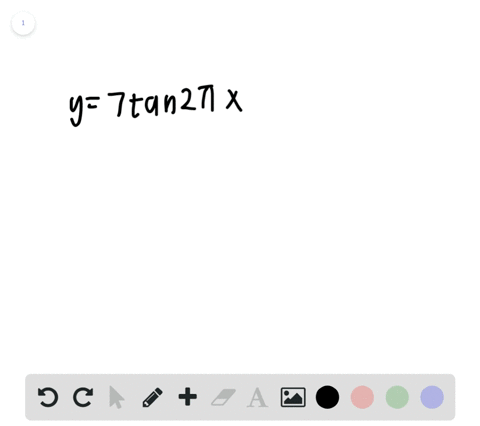🎉 The Study-to-Win Winning Ticket number has been announced! Go to your Tickets dashboard to see if you won! 🎉View Winning Ticket### Period In Exercises $49-52,$ find the period of t…

00:37University of Southern California
Problem 48

# Period and Amplitude In Exercises $45-48,$ determine the period and amplitude of each function.$$y=\frac{2}{3} \cos \frac{\pi x}{10}$$

## Discussion

You must be signed in to discuss.

## Video Transcript

problem. 48 asks us to find the amplitude in the period of the following function. Why equals 2/3 co sign of pie, 10th X Now we're looking to find the period and amplitude of a given function specifically co sign. We can use this general equation to help identify those two values. So first, to find the period, all you have to do is look at what's contained inside of our function. So we look at this be value and we know of the period of co sign equals to pie divided by the absolute value of whatever that is now looking over at our equation. Over here we see that r b value his pie 10th. So what we can do is we can say the period's gonna equal to pi. Divided by pi tense, I dropped the absolute value signed because pie 10th is positive. Then we see the two pi divided by by tens is the same as two pi times 10 over pi pies cancel out and we're left with the period equaling 20. Now the amplitude is generally easier to find. All we have to do is look at this a value that's on the outside of our tricking a metric function, and the amplitude is just going to be the absolute value of a. So if we look at our given function, we find that 2/3 is on the outside and so the amplitude is simply going to be 2/3.